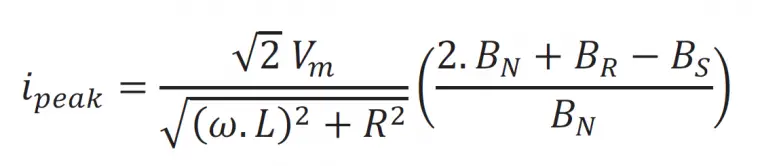# Transformer Inrush Current Calculator With Formula

### Inrush Current calculator:

Enter the input voltage in volts, DC resistance of the winding then press the calculate button to get inrush current in amps.

 Enter Input Voltage: V Enter Winding resistance: ohm Result – Inrush Current: A

### What is inrush current:

Inrush current is nothing but a maximum flow of current at that time of starting electrical equipment. The current flows a few seconds only. The inrush current causes equipment rapid heating, puncturing the coils, dames the insulation and in worst case equipment failure.

The inrush current for the rotating equipment will be higher than the static equipment. Example. For the same capacity of motor takes higher current than the transformers.

### Inrush current calculations:

Approximately inrush current I(peak) is equal to the ratio between the root 2 times of maximum applied voltage to DC winding resistance. This calculation gives you the approximate value of the inrush current to understand.

I(peak) = 1.414 Vm / R(ohms) Amps

To get the accurate value of the inrush current use the below-mentioned formula,Vm – maximum applied voltage in volts; L – air core inductance of the transformer in Hendry; R – total dc resistance of the transformer in Ohms; BN – normal rated flux density of the transformer core in weber per meter square; BR – remnant flux density of the transformer core in weber per meter square; BS – saturation flux density of the core material in weber per meter square.

Example:

A 100kVA, 440V transformer has a DC winding resistance of 0.45 ohms, Calculate the inrush current of the transformer.

Apply our formula,

I(peak) = 1.414 x 440 / 0.45

Inrush current in Amps = 1382 Amps

At the same time full load current of the transformer

I(A) = kVA *1000 / Voltage

Full load current in Amps = 1000 * 100 / 440 = 227 Amps

Hence inrush current is equal to the 6 times of the full load current.

Reference: